# The ROBUSTREG Procedure

## Details: ROBUSTREG Procedure

Subsections:

This section describes the statistical and computational aspects of the ROBUSTREG procedure. The following notation is used throughout the section.

Let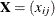denote an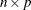matrix, let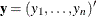denote a given n-vector of responses, and let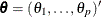denote an unknown p-vector of parameters or coefficients whose components are to be estimated. The matrixis called the design matrix. Consider the usual linear model,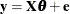where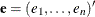is an n-vector of unknown errors. It is assumed that (for a given) the components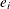ofare independent and identically distributed according to a distribution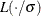, where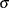is a scale parameter (usually unknown). Often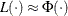, the standard normal distribution function. The vector of residuals for a given value of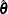is denoted by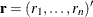, and the ith row of the matrixis denoted by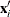.